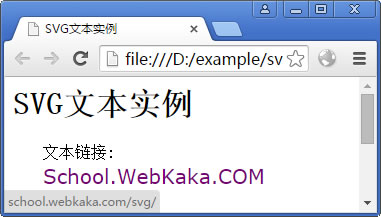# SVG Text（文本）

<text>元素是用来绘制文本的。

## 语法

`<text  x="x轴坐标"  y="y轴坐标"  dx="长度"  dy="长度"   rotate="数字"  textlength="长度"  lengthAdjust="spacing" ></text>`

## 属性

Sr.No. 属性与描述
1 x − x轴坐标.
2 y − y轴坐标.
3 dx − 偏移x轴长度.
4 dy − 偏移y轴长度.
5 tspan多行文本.
6 textlength文本长度.
8 rotate转动角度.

## 实例

SVG_text.html
`<html>   <title>SVG文本</title>   <body>      <h1>SVG文本实例</h1>      <svg width="300" height="200">         <g>            <text x="30" y="12" >Text: </text>            <text x="30" y="30" fill="rgb(121,0,121)">School.WebKaka.COM</text>         </g>       </svg>   </body></html>`

## 输出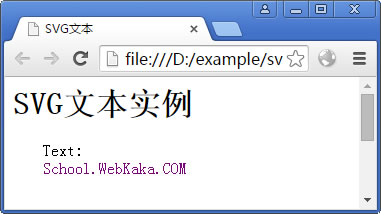SVG文本实例

## 多行文本

SVG_Multline_Text.html
`<html>   <title>SVG文本实例</title>   <body>      <h1>SVG文本实例</h1>      <svg width="300" height="200">         <g>            <text x="30" y="12" >多行文本: </text>            <text x="30" y="30" fill="rgb(121,0,121)">School.WebKaka.COM            <tspan x="30" y="50" font-weight="bold">你的网上学习园地.</tspan>            <tspan x="30" y="70">所有教程都是免费的.</tspan>            </text>         </g>      </svg>   </body></html>`

## 输出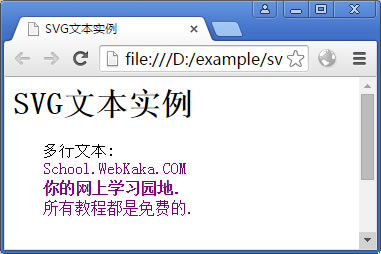SVG文本实例（多行文本）

`<text x="30" y="12"  dy="2">多行文本: </text>`

## 文本长度

SVG_textlength.html
`<html>   <title>SVG文本</title>   <body>      <h1>SVG文本实例</h1>      <svg width="300" height="200">         <g>            <text x="30" y="12" dy="2">文本默认长度 </text>            <text x="30" y="38" textlength="200" fill="rgb(121,0,121)">文本长度设为200</text>            <text x="30" y="60" fill="rgb(0,0,0)">School.WebKaka.COM</text>         </g>       </svg>   </body></html>`

## 输出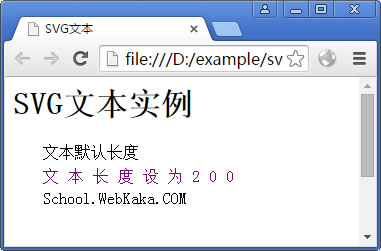SVG文本实例（文本长度）

## 转动文本

SVG_Rotate_Text.html
`<html><title>SVG文本</title><body><h1>SVG文本实例</h1><svg width="300" height="130">   <g>      <text x="30" y="12" >转动文本: </text>      <text x="30" y="30"fill="rgb(121,0,121)"       transform="rotate(30 20,40)" >School.WebKaka.COM</text>   </g></svg></body></html>`

## 输出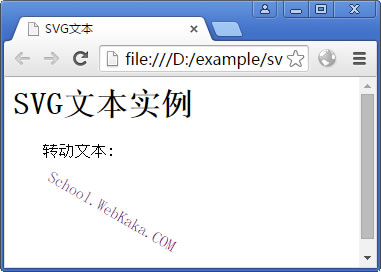SVG文本实例（转动文本）

`<html>   <title>SVG文本实例</title>   <body>      <h1>SVG文本实例</h1>      <svg width="300" height="200">         <g>            <text x="30" y="10" dy="4">文本链接: </text>            <a xlink:href="http://school.webkaka.com/svg/" target="_blank">               <text font-family="Verdana" font-size="20"  x="30" y="40"                fill="rgb(121,0,121)">School.WebKaka.COM</text>            </a>         </g>      </svg>   </body></html>`